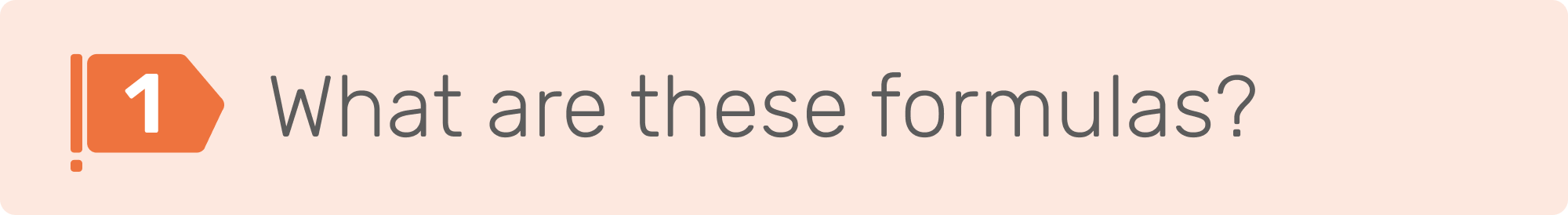Random & Random Integer
Share
ExploreRandom & Random Integer#Random & Random IntegerRandom & Random IntegerFormulas: Random() and RandomInteger()
Category: Math
`⁠⁠``Random()`
Returns a random number
or stated otherwise...
Hey Coda, will you please generate a random number below 1 (.99 and below) for me? I may or may not want you to update this number for every doc edit.
`⁠⁠``RandomInteger()`
Returns random number between two numbers
put a bit simpler...
Hey Coda, will you generate a random number that falls between these two numbers. I may or may not want you to update this number for every doc edit.

Random() and RandomInteger() share one parameter. RandomInteger() has two additional parameters:
`⁠⁠``UpdateContinuously`
`Random(UndateContinuously)`
`Whether or not to have the random value update every time an edit is made on the doc; True or False.`
`⁠⁠``High`
`RandomInteger(High, Low, UpdateContinuously)`
`The highest possible number that can be genearated.`
`⁠⁠``Low`
`RandomInteger(High, Low, UpdateContinuously)`
`The lowest possible number that can be generated.`Random() and RandomInteger() will likely be used a bit less than other formulas. One major difference between the two is that Random() returns random numbers between 0 and .99, while RandomInteger() will return any whole number between the numbers you designated in the High and Low parameters.

Give Me New Numbers!Random()
0.35555942144966596RandomInteger()
159

Notice how the button changes every time you hit the button...that’s the UpdateContinuously parameter at work! That parameter is marked as “True” in both instances. But what happens when it’s false? Here’s the same table again, but this time with an option to toggle that parameter!

Give Me New Numbers!
UpdateContinuously = False
UpdateContinuously = TrueRandom()
0.6772235902237118RandomInteger()
678

Let’s move to our activities to see some use cases for these formulas!Practice: Try out the basics of Random() and RandomInteger()
DoneUse the new formulas to fill columns in a sample database
DoneChallenge: Apply your knowledge to create a fun game!
Done
Share